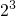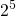# Complexity Explorer Santa Fe Institute

Your progress is not being saved! Enroll now or log in to track your progress or submit homework.## Introduction to Information Theory

• Measuring Information: Bits
• Adding Up Bits
• Using Bits to Count and Label
• Physical Forms of Information
• Fundamental Formula of Information
• Computation and Logic: Information Processing
• Mutual Information
• Communication Capacity in a Noisy Channel
• Shannon's Coding Theorem
• Homework Solutions

#### 1.3 Using Bits to Count and Label » Quiz 1 Solutions

Question 1:

Remember that 1 bit can by itself represent 2 states (which can be labelled as 0 and 1).

2 bits can represent 4 states, corresponding to the pairs (0, 0), (0, 1), (1, 0), and (1, 1).

We express this more conveniently by using exponentiation. The total number of states that a sequence of n bits can represent is 2n , i.e., the number of states of a single bit (2) raised to the number of bits (n).

It follows that the number of states that 3 bits can represent is=8. Similarly, the number of states that 5 bits can represent is= 32.

Question 2:

We know that a single bit can represent two states, two bits can represent four states, and three bits can represent 8 states. Thus, the answer to question 2 is 3. (see the answer to Question 1 for more details)

More generally, the number of states that n bits can represent is 2n. Given any number of states S, the number of bits needed to represent S states is specified by the number n which solves 2n = S. We can use the “logarithm” function, which is the opposite of exponentiation, to find the n that correspond to a given S.

The logarithm of some number S in “base” b is defined to be that number n which satisfies bn = S, and can be written as n = logb S.  Thus, the logarithm in base 2 of 8 is 3, which we write as log2 8 = 3, the logarithm of 32 in base 2 is 5, which we write as log2 32 = 5, and so on.

Question 3:

The correct answer is 300.  There are two ways to arrive at this answer.

First, note that the number of bits necessary to represent some number of states S is log2 S.  By using a digital calculator, we can compute that log2 1090 ≈ 298 ≈ 300 (this assumes the calculator can handle very large numbers like 1090).

There is another way to arrive at this answer, however. Remember the exponentiation rule that (ca)b = ca*b.  Then, note that we can write 1090 = (103)30. Using the fact that 103 ≈ 210, we can combine to write 1090 = (103)30 ≈ (210)30 = 210*30 = 2300. Finally, we know that 2300 states can be represented by 300 bits.

Question 4:

As stated, a single nucleotide can represent 4 states, written A, C, G, or T. Two nucleotides can represent 16 states: (A,A), (A,C), (A,G), (A,T), (C,A), (C,C), (C,G), (C,T), (G,A), (G,C), (G,G), (G,T), (T,A), (T,C), (T,G), and (A,T).  Using the same reasoning as in the answer to Question 1, the number of sequences that can be represented by n nucleotides is 4n, i.e., the number of states of a single nucleotide (4) raised to the number of nucleotides (n).

Since 3 billion is equal to 3,000,000,000, the number of sequences that can be represented by a genome with 3 billion nucleotides is 43,000,000,000.